• Slides: 12Column Addition Column addition involves: • Recording numbers in place-value columns; • Adding in place-value columns; and • Making and moving groups of 1 s, 100 s, and so on. EverydayColumn Addition Solve: 379 + 562 We set up the problem by writing the numbers in their place-value columns. H = hundreds T = tens O = ones H 3 +5 T 7 6 Everyday O 9 2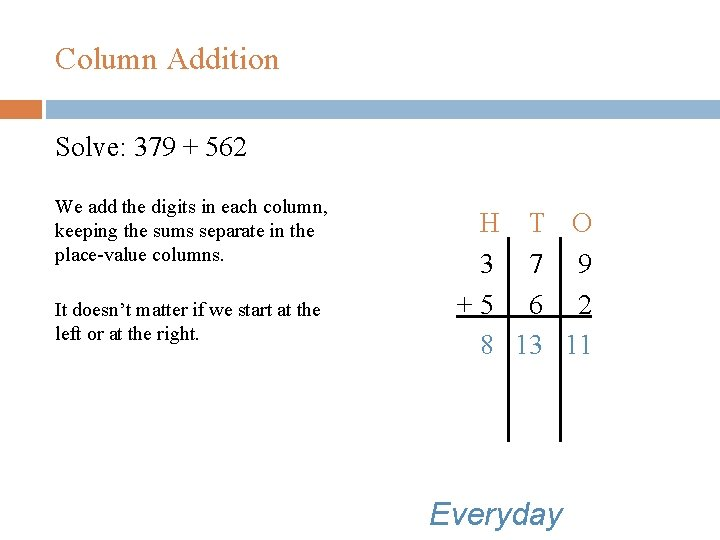Column Addition Solve: 379 + 562 We add the digits in each column, keeping the sums separate in the place-value columns. It doesn’t matter if we start at the left or at the right. H T O 3 7 9 +5 6 2 8 13 11 Everyday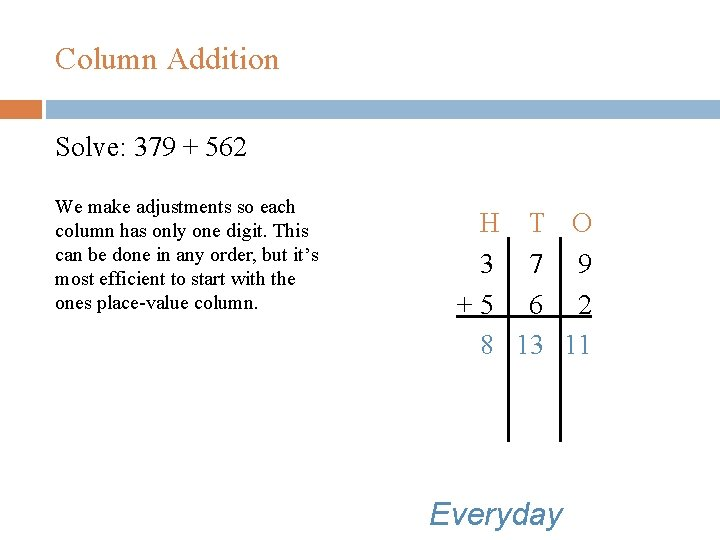Column Addition Solve: 379 + 562 We make adjustments so each column has only one digit. This can be done in any order, but it’s most efficient to start with the ones place-value column. H T O 3 7 9 +5 6 2 8 13 11 Everyday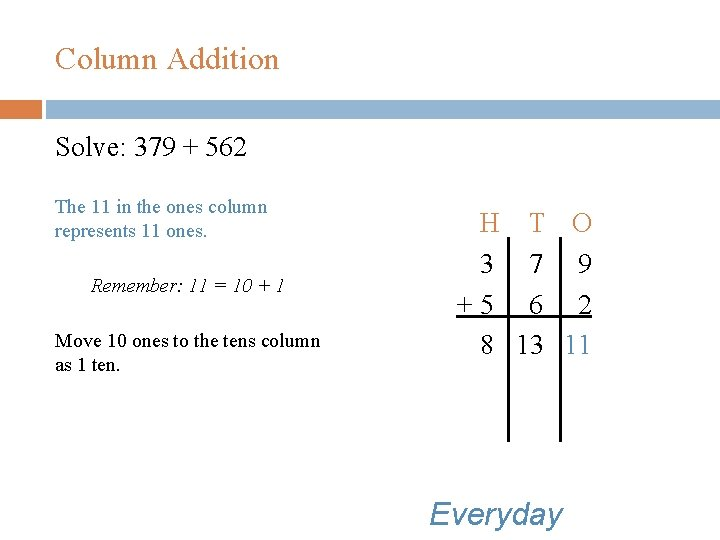Column Addition Solve: 379 + 562 The 11 in the ones column represents 11 ones. Remember: 11 = 10 + 1 Move 10 ones to the tens column as 1 ten. H T O 3 7 9 +5 6 2 8 13 11 Everyday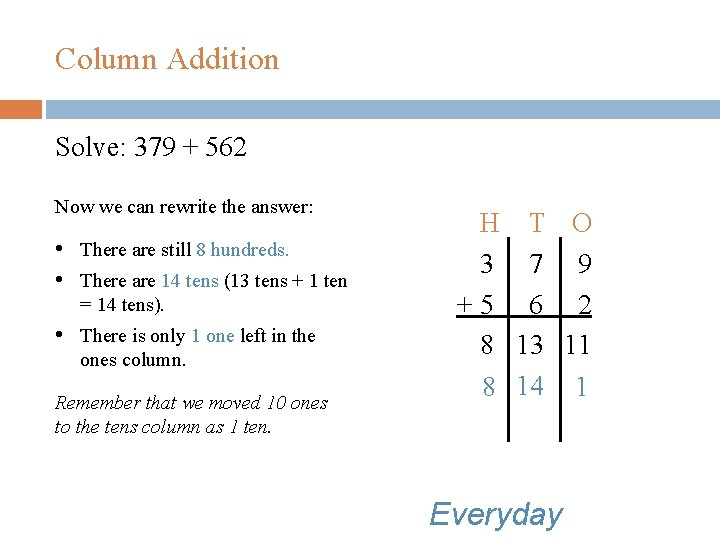Column Addition Solve: 379 + 562 Now we can rewrite the answer: • There are still 8 hundreds. • There are 14 tens (13 tens + 1 ten = 14 tens). • There is only 1 one left in the ones column. Remember that we moved 10 ones to the tens column as 1 ten. H T O 3 7 9 +5 6 2 8 13 11 8 14 1 Everyday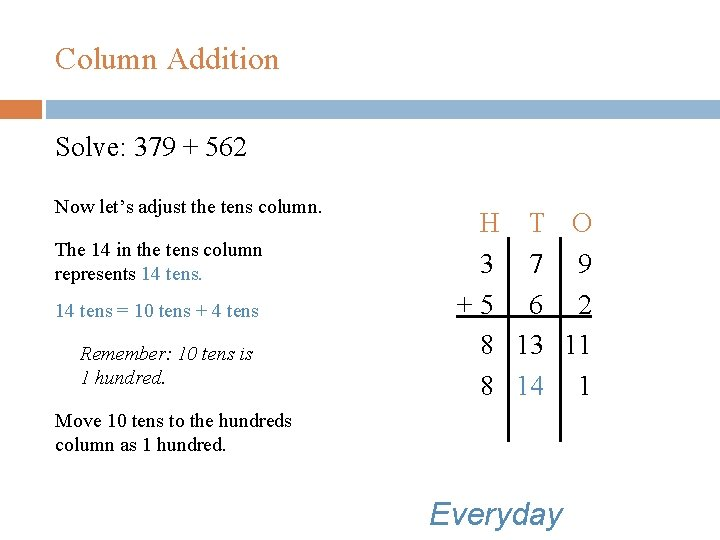Column Addition Solve: 379 + 562 Now let’s adjust the tens column. The 14 in the tens column represents 14 tens = 10 tens + 4 tens Remember: 10 tens is 1 hundred. H T O 3 7 9 +5 6 2 8 13 11 8 14 1 Move 10 tens to the hundreds column as 1 hundred. Everyday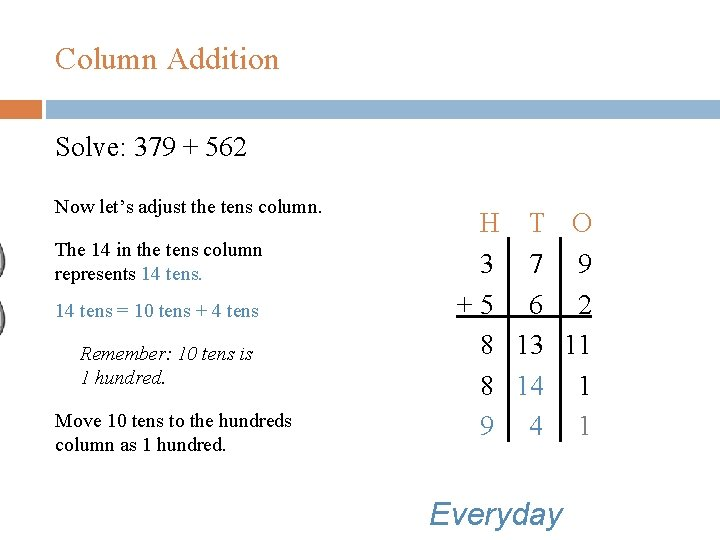Column Addition Solve: 379 + 562 Now let’s adjust the tens column. The 14 in the tens column represents 14 tens = 10 tens + 4 tens Remember: 10 tens is 1 hundred. Move 10 tens to the hundreds column as 1 hundred. H T O 3 7 9 +5 6 2 8 13 11 8 14 1 9 4 1 Everyday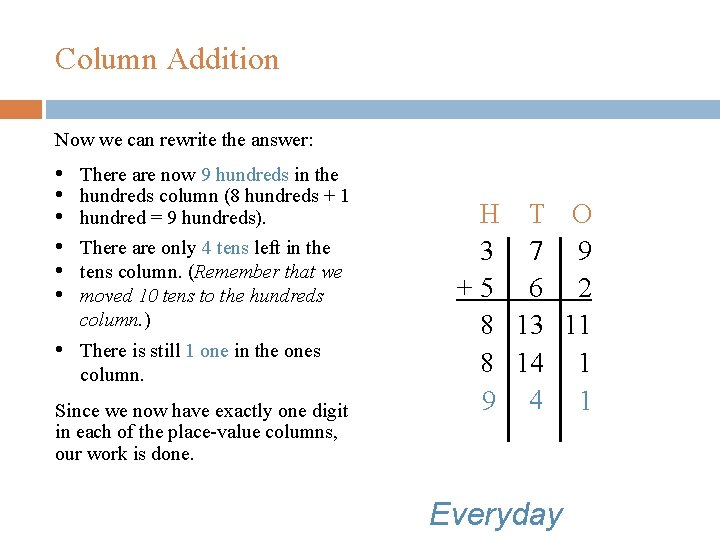Column Addition Now we can rewrite the answer: • • • There are now 9 hundreds in the hundreds column (8 hundreds + 1 hundred = 9 hundreds). There are only 4 tens left in the tens column. (Remember that we moved 10 tens to the hundreds column. ) • There is still 1 one in the ones column. Since we now have exactly one digit in each of the place-value columns, our work is done. H T O 3 7 9 +5 6 2 8 13 11 8 14 1 9 4 1 Everyday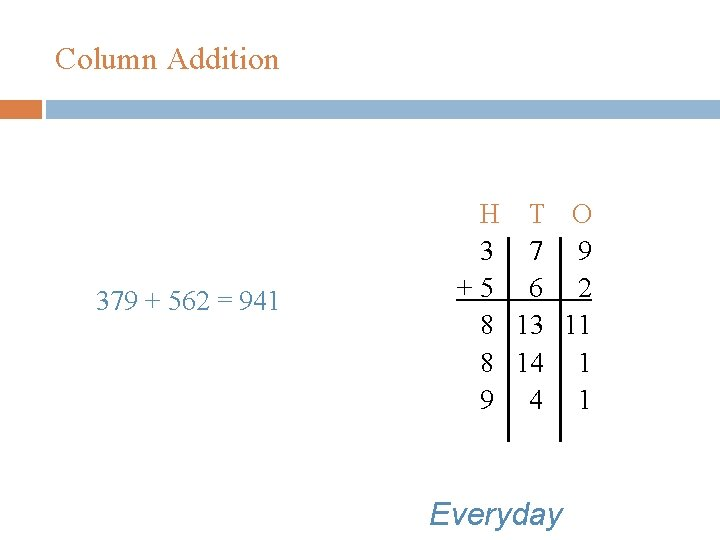Column Addition 379 + 562 = 941 H T O 3 7 9 +5 6 2 8 13 11 8 14 1 9 4 1 Everyday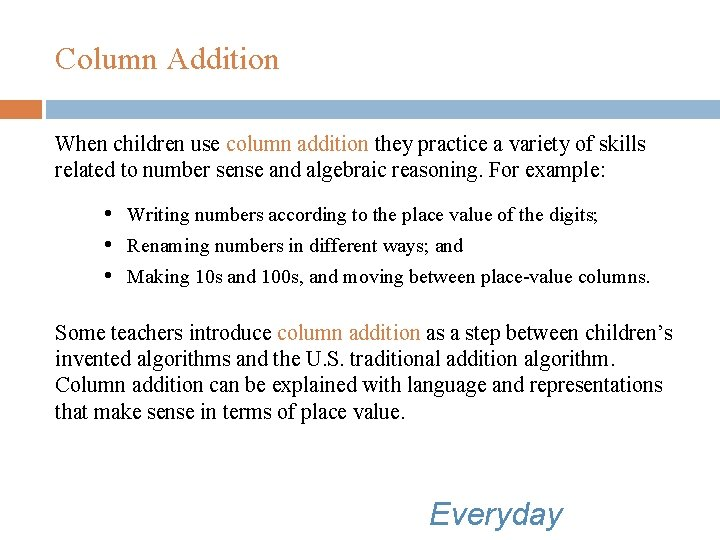Column Addition When children use column addition they practice a variety of skills related to number sense and algebraic reasoning. For example: • Writing numbers according to the place value of the digits; • Renaming numbers in different ways; and • Making 10 s and 100 s, and moving between place-value columns. Some teachers introduce column addition as a step between children’s invented algorithms and the U. S. traditional addition algorithm. Column addition can be explained with language and representations that make sense in terms of place value. Everyday